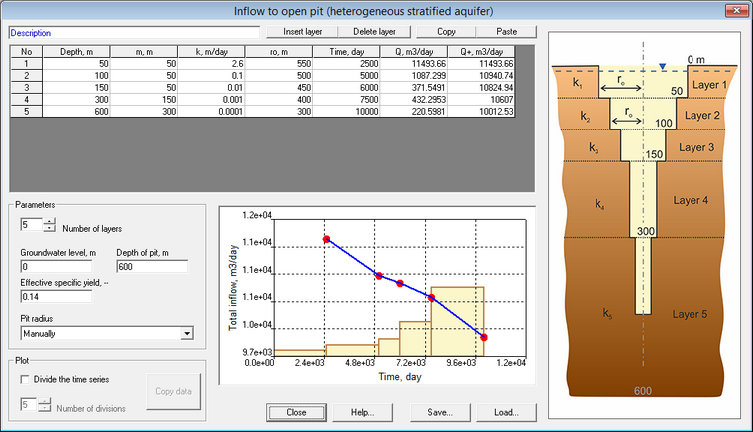﻿ Analytical modeling (AMWELLS) > Pit dewatering > Pit Inflow > Heterogeneous stratified aquifer

# Heterogeneous stratified aquifer

Navigation:  Analytical modeling (AMWELLS) > Pit dewatering > Pit Inflow >

# Heterogeneous stratified aquifer

This dialog window calculates inflows to an open pit in the aquifer, where permeability decreases with increasing depth. Hydrogeological profile is approximated by layers, where hydraulic conductivity is decreasing in each successive layer from the shallowest to the deepest. The dialog window is accessible for unconfined aquifers and can be launched from the window “Pit inflow” by the button “Heterogeneous stratified aquifer”

The solutions are accurate when the difference between layer hydraulic conductivities is significant, at least one order of magnitude. For smoother decreases in hydraulic conductivity profile, it is recommended to use the “Pit inflow” main window and conduct calculations separately for different stages of pit excavation using effective parameters (Menu Hydrogeologist workbench > Estimation of parameters > Effective values).Explanatory notes

 Field above the table Text field for project info Table The table in this dialog window contains input data for pit dimensions, hydraulic conductivity, mine schedule and outputs – calculated groundwater inflows. Each row corresponds to one layer. When pit excavation is above layer top, the layer is shadowed grey and is not included in calculations. When a layer is partially penetrated by excavation, the layer is highlighted in blue and is included in calculations only to its penetrated depth. Column N0 – sequential number of layers Column “Depth” – depth of the base of each layer, measured from the ground surface. The value in this column has to be higher for each subsequent layer Column “m” – layer thickness. The values in this column are automatically calculated based on the column “Depth”. For the layer with groundwater table, its initial saturated thickness is calculated. Unsaturated layers should not be included as separate units, they should be included in the layer containing the initial water table. The topmost layer should always be set so it includes the initial water table. Column "k" –  layer hydraulic conductivity, m/day Column "ro", "Area" or "Perimeter" – determines effective radius of the pit. Depending on the selection in the list “Pit radius”, either the radius of the pit or the pit’s area or perimeter have to be input in this column. When the area or the perimeter values are input in this column, the radius is calculated automatically. Column "Time" – time (days) based on the pit excavation schedule. This time is used for calculation of radius of influence as well as groundwater inflow calculations and plots. The time entered corresponds to the time since the beginning of operations and not the operations time in each layer. The time should always increase with each successive layer. Column "Q" – calculated groundwater inflow into the pit for the layer for the time determined in Column “Time”. The inflow value is used to calculate values in the Column "Q+" Column "Q+" – total groundwater inflow into the pit for the time determined in Column “Time” Button "Insert layer" Adds a layer to the cursor location Button "Delete layer" Deletes layer at the cursor location Button "Copy" Copies data from the Table to the clipboard Button "Paste" Pastes data from the clipboard on the Table starting from the cursor location Frame "Parameters" Information about hydrogeological profile and pit dimensions. When the parameters are amended in this frame, groundwater inflow parameters are recalculated Field "Number of layers" Number of layers (between 2 and 99) with different hydraulic conductivity Field "Groundwater level" Depth of groundwater table. The minimum value in this field is 0, it corresponds to the water table at ground level Field "Depth of pit" Depth of pit excavation Field "Effective specific yield" Specific yield that is used for calculation of radius of influence. Its effective value is usually similar to the specific yield of the upper layer. This field is inactive for steady state conditions List "Pit radius" Selection of methods to calculate the radius of influence: manually, area-based or perimeter-based. Calculation formulas see in section "Pit inflow" Frame "Plot" Inflow plot settings Option "Divide the time series" With the selected option, time periods are divided into intervals and inflow is recalculated for each interval. The number of intervals is determined by the Field “Number of divisions”. For steady state conditions, inflows are time-independent Field "Number of divisions" Number of divisions (between 1 and 9) for each time interval – the same for all intervals Button "Copy data" Plot is saved on the clipboard in the table form. This button is accessible with the checked option “Divide the time series” Plot Total pit inflow (Column "Q+" of the Table) versus time (Column "Time" of the Table) Bar diagram shows кудфешму layer depths for the times, when inflows were calculated Button "Save" Saves all data and plots in the dialog window in the internal ANSDIMAT format (.ATP) for future use Button "Load" Loads file with previously saved data for this dialog window (.ATP file)